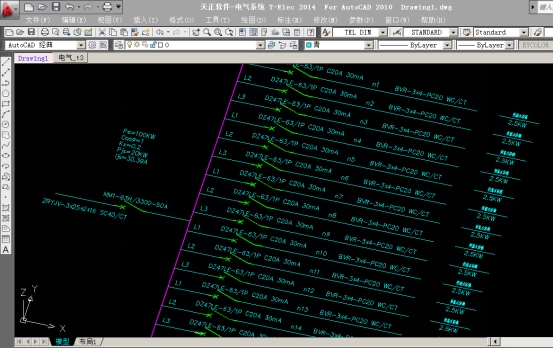Pe=2.5KW×40=100kw

Kx=0.2

Cosφ=1（纯阻性负载）

Pjs=100×0.2=20KW

Ijs=20÷0.38÷1.732÷1=30.39A